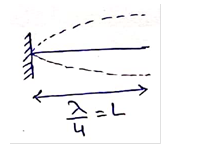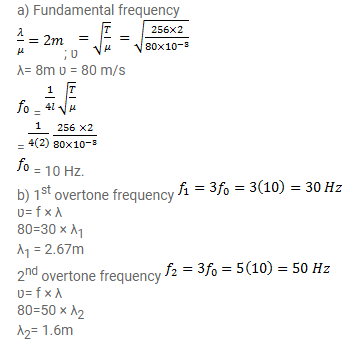# A 2.00m long rope, having a mass of 80g,Question:

A $2.00 \mathrm{~m}$ long rope, having a mass of $80 \mathrm{~g}$, is fixed at one end and is tied to a light string at the other end. The tension in the string is $256 \mathrm{~N}$

a. Find the frequencies of the fundamental and the first two overtones.

b. Find the wavelength in the fundamental and the first overtones.

Solution: